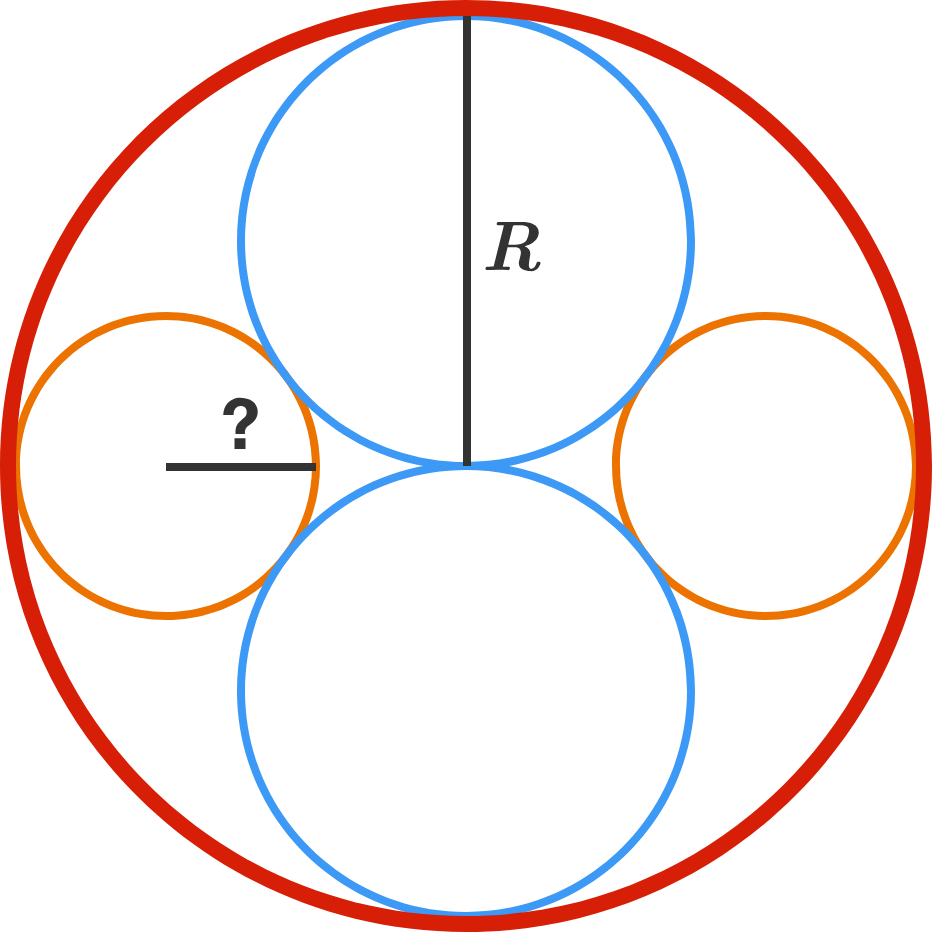# Circular Double Twins

Geometry Level 1There are two identical blue circles and two identical orange circles arranged symmetrically in a larger red circle, as shown. The circles are tangent to each other where they touch.

If the radius of the red circle is $R,$ then what is the radius of one of the orange circles?

Hint: Try finding a right triangle and applying the Pythagorean theorem.

×